# Mozgostroje

## Guess what?! Another Analysis of the Schnall-Johnson Data

25 Jun 2014

[]

By now basically everyone (here, here, here, here and here, and there is likely even more out there) who writes a blog and knows how to do a statistical analysis has analysed data from a recent replication study and from the original study (data repository is here).

The study consists of two experiments. Let's focus on Experiment 1 here. The experiment tests a treatment and a control group. The performance is measured by six likert-scale items. The scale of each item has 9 levels. All responses are averaged together and we obtain a single composite score for each group. We are interested whether the treatment works, which would show up as a positive difference between the composite score of the treatment and the control group. Replication study did the same with more subjects.

Let's perform the original analysis to see why this dataset is so "popular".

In :
%pylab inline
import pystan
from matustools.matusplotlib import *
from scipy import stats
import warnings
warnings.filterwarnings("ignore")

Populating the interactive namespace from numpy and matplotlib

In :
il=['dog','trolley','wallet','plane','resume',
'kitten','mean score','median score']
D[:,1]=1-D[:,1]
Dtemp=np.zeros((D.shape,D.shape+1))
Dtemp[:,:-1]=D
Dtemp[:,-1]=np.median(D[:,2:-2],axis=1)
D=Dtemp
DS=D[D[:,0]==0,1:]
DR=D[D[:,0]==1,1:]
DS.shape

Out:
(40, 9)
In :
plt.figure(figsize=(8,3))
ax=plt.subplot(1,2,1)
dts=[DS[DS[:,0]==0,-2],DS[DS[:,0]==1,-2],
DR[DR[:,0]==0,-2],DR[DR[:,0]==1,-2]]
for k in range(len(dts)):
plotCIttest1(dts[k],x=k)
plt.grid(False,axis='x')
plt.ylabel('mean score')
ax.set_xticks(range(len(dts)))
ax.set_xticklabels(['OC','OT','RC','RT'])
plt.xlim([-0.5,len(dts)-0.5])
ax=plt.subplot(1,2,2)
print np.round(plotCIttest2(dts,dts,x=0,alpha=0.1),3)
print np.round(plotCIttest2(dts,dts,x=1,alpha=0.1),3)
ax.set_xticks([0,1]);plt.ylabel('score difference')
ax.set_xticklabels(['OT-OC','RT-RC'])
plt.grid(False,axis='x')
plt.xlim([-0.5,1.5]);

[ 0.825  1.537  0.113  1.113  0.537]
[ 0.01   0.267 -0.247  0.115 -0.095]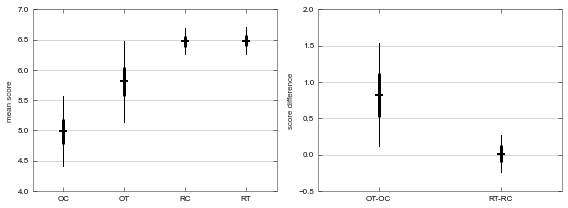Legend: O - original study, R - replication, C - control group, T - treatment group

In the original study the difference between the treatment and control is significantly greater than zero. In the replication, it is not. However the ratings in the replication study are overall higher. The author of the original study therefore raised a concern that no difference was obtained in replication because of the presence of ceiling effects.

How do we show that there are ceiling efects in the replication? The authors and bloggers presented various arguments that support some conclusion (mostly that there are no ceiling effects). Ultimately ceiling effects are a matter of degree and since no one knows how to quantify them the whole discussion of the validity of the conclusions from the replication study is heading into an inferential limbo.

My point here is that if the analysis computed the proper effect size - the causal effect size, we would avoid these kinds of arguments and discussions. Actually, this is fairly easy to see. If we interpret T-C estimate causally then it says that by giving people the treatment we increase their score by 0.83 points on average. So when their base score is 9.5 points we predict that the treatment will increase it to 10.33. This however can't happen because the scale goes only from 1 to 10. A quick fix is to say that the resulting score will be a minimum of the raw score and the upper ceiling value 10. This implies that a group with a base score 9.1 will end up with a score 9.93 which is again implausible. We need some function that maps from $[-\infty,\infty]$ (logit scale) into $[1,10]$ in a continuous fashion. The logistic function is the usual choice. (Probit function is also popular.) This is what it looks like:

In :
x=np.linspace(-10,10,101)
def mylgs(x): return 9/(1+np.exp(-x))+1

for k in [1,3,7,9]:
plt.plot([k,k],[1,mylgs(k)],'k--');
plt.plot([k,-10],[mylgs(k),mylgs(k)],'k--');
plt.plot(x,mylgs(x),'r',lw=2);
plt.grid(False,axis='x')
plt.gca().set_xticks(range(-10,10));
plt.ylabel('Raw score')
plt.xlabel('Logit score');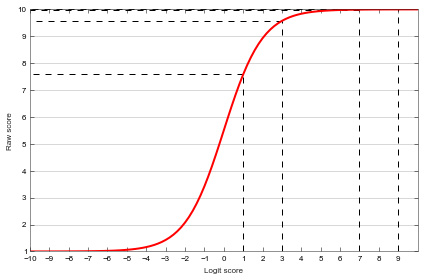We measure and report the effect size on the logit scale. To obtain precise prediction we can always transform into raw score. Then a claim that a treatment increases logit score, say, by 2 units does not produce any counter-intuitive results. This is illustrated above for two groups with base logit score of 1 and 7 respectively. The increase by 2 units on the logit scale leads to big jump in the raw score in the former case while in the latter case the raw score remains the same.

The author of the original study voiced concern that the ceiling effects may not be detectable in the composite score and we should focus on individual items. Let's look at the distribution of the scores of the individual items in the original study and in the replication.

In :
def plotComparison(A,B,stan=False):
plt.figure(figsize=(8,16))
cl=['control','treatment']
x=np.arange(11)-0.5
if not stan:assert A.shape==B.shape
for i in range(A.shape-1):
for cond in range(2):
plt.subplot(A.shape-1,2,2*i+cond+1)
a=np.histogram(A[A[:,0]==cond,1+i],
bins=x, normed=True)
plt.barh(x[:-1],-a,ec='w',height=1)
if stan: a=[B[:,i,cond]]
else:
a=np.histogram(B[B[:,0]==cond,1+i],
bins=x, normed=True)
plt.barh(x[:-1],a,ec='w',fc='g',height=1)
plt.xlim([-0.7,0.7]);
plt.gca().set_yticks(range(10))
plt.ylim([-1,10]);
if not i: plt.title(cl[cond])
pref=['','item: '][int(i<6)]
if not cond: plt.ylabel(pref+il[i],size=12)
if not i and not cond:
plt.legend(['original','replication'],loc=4);
plotComparison(DS,DR)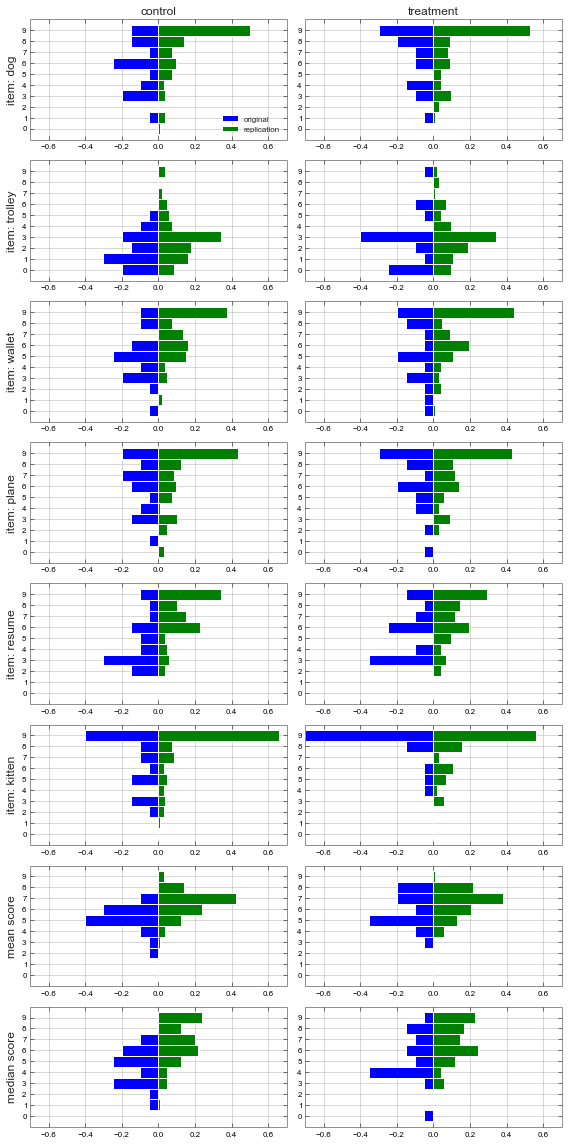We see that RC shows higher scores than OC on all items. We see also that the response distribution is multimodal (see the resume item). Furthermore the outcome variable is discrete. In contrast, the logistic distribution is unimodal and continuous.

As a consequence we need a better model. We will use ordered logit model which can be seen as an extension of the simple logit model. The probability of a response $k=1,\dots,K$ (in our case $K=10$) is $P(x=k) = \mathrm{logistic}(\beta-c_k)-\mathrm{logistic}(\beta-c_{k-1})$. $c_0=-\infty$ and $c_K=\infty$. The remaining coefficients $c_i$ are estimated from the data. $\beta$ is the item difficulty. $c_k$ can be interpreted as the response thresholds. For instance if $\beta$ equals $c_5$ then a response higher than 5 is given half of the times. The coefficients $\beta, c_i$ give only ordinal information and we may wish to fix one of them to a constant value (say $\beta=0$). If we have two groups we can fix the difficulty for the control group to zero and estimate the difficulty in the treatment group. This quantity then gives the causal effect size.

As with the logistic model above, the ordered logit accounts for ceiling effects in accord with our intuition. We already saw one aspect of how this works - big differences in logit score get squashed into small differences in the raw score. How does this work in the other way? At first blush small differences in the raw score at the ceiling should be transformed into big differences in the logit score. However, while the raw score differences quickly get small at the ceiling the estimation error does not decrease so quickly. As a consequence we get a poor signal-to-noise ratio at the ceiling and what we obtain is an uncertain logit-score estimate. Intuitively, this is what we would expect from a ceiling effect - in its presence it is difficult to say whether a reliable difference between two scores exists and what its magnitude is.

I hope you are appetized now, so let's see what the ordered logit model tells us.

To make it precise we will use the following model.

$y_{n,m}^g \sim \mathrm{orderedLogit}(\beta_m +\delta_g,c)$

$y_{n,m}^g$ is the response of subject $n$ to item $m$, i.e. a value in $[1,10]$. $g$ indexes the four groups which are of main interest to us. These are OC,OT,RC and RT. $\beta_m$ gives the difficulty for each of the six items. $c$ is the threshold vector. $\delta_g$ gives the ability of each group. We fix $\delta_{\mathrm{oc}}$ to zero.

In :
model='''
data {
int<lower=2> K;
int<lower=0> N;
int<lower=1> M;
// y - response of subject n to item m
int<lower=1,upper=K> y[N,M];
// x - first column 0=original,1=replication
// second column 0=control,1=treatment
int x[N,2];
}
parameters {
vector[M-1] bbeta;
real dd;
ordered[K-1] c;
}
transformed parameters{
vector[M] beta;
for (m in 1:M){
if (m==1) beta[m]<-0.0;
else beta[m]<-bbeta[m-1];
}}
model {
for (k in 1:(K-1)) c[k]~ uniform(-100,100);
for (m in 1:M){
for (n in 1:N) y[n,m] ~ ordered_logistic(-beta[m]
+dd*x[n,1]*(1-x[n,2]) // RC
+dd*x[n,2]*(1-x[n,1]) // OT
+dd*x[n,1]*x[n,2], c); // RT
}}
'''
sm5=pystan.StanModel(model_code=model)

In :
dat = {'y':np.int32(D[:,2:8])+1,'K':10,'M':6,
'x':np.int32(D[:,[0,1]]),'N':D.shape }
fit6 = sm5.sampling(data=dat,iter=5000, chains=4,
thin=2,warmup=100,njobs=4,seed=4)
print pystan.misc._print_stanfit(fit6,
pars=['dd','bbeta','c'],digits_summary=2)
saveStanFit(fit6,'fit6')

Inference for Stan model: anon_model_49eb9ff852a47d3536e8cefa052d1f67.
4 chains, each with iter=5000; warmup=100; thin=2;
post-warmup draws per chain=2450, total post-warmup draws=9800.

mean se_mean   sd 2.5%  25%  50%  75% 97.5% n_eff Rhat
dd    0.67     0.0 0.23 0.21 0.51 0.67 0.82  1.132223.0  1.0
dd    1.23     0.0 0.18 0.88 1.11 1.23 1.35  1.582176.0  1.0
dd    1.21     0.0 0.18 0.85 1.09 1.21 1.33  1.562168.0  1.0
bbeta 3.41     0.0 0.19 3.05 3.28 3.41 3.53  3.782279.0  1.0
bbeta 0.45     0.0 0.16 0.13 0.34 0.45 0.56  0.772492.0  1.0
bbeta 0.24     0.0 0.17-0.08 0.13 0.25 0.36  0.572576.0  1.0
bbeta 0.62     0.0 0.16  0.3 0.51 0.62 0.72  0.942441.0  1.0
bbeta-0.56     0.0 0.17 -0.9-0.68-0.56-0.45 -0.222483.0  1.0
c    -4.44    0.01 0.26-4.95-4.61-4.43-4.26 -3.931909.0  1.0
c    -3.42    0.01 0.23-3.87-3.58-3.42-3.27 -2.982006.0  1.0
c    -2.53     0.0 0.21-2.96-2.67-2.52-2.38 -2.122014.0  1.0
c    -1.22     0.0  0.2-1.61-1.35-1.21-1.09 -0.842005.0  1.0
c    -0.83     0.0  0.2-1.22-0.96-0.82-0.69 -0.452025.0  1.0
c    -0.32     0.0 0.19 -0.7-0.44-0.31-0.18  0.062017.0  1.0
c     0.37     0.0 0.19-0.01 0.24 0.37  0.5  0.752039.0  1.0
c     0.78     0.0  0.2 0.39 0.64 0.78 0.91  1.162042.0  1.0
c     1.25     0.0  0.2 0.86 1.11 1.25 1.38  1.632039.0  1.0

Samples were drawn using NUTS(diag_e) at Tue Jun 24 15:41:36 2014.
For each parameter, n_eff is a crude measure of effective sample size,
and Rhat is the potential scale reduction factor on split chains (at
convergence, Rhat=1).

INFO:pystan:NOW ON CHAIN 0
INFO:pystan:NOW ON CHAIN 3
INFO:pystan:NOW ON CHAIN 1
INFO:pystan:NOW ON CHAIN 2


The estimated values are displayed below. Reacall that the zero on the vertical axis shows the difficulty of the dog item and the OC group. We see that the trolley item is most difficult while the kitten item is easiest. The estimates of group ability show identical pattern as the raw score estimates. In particular, there is a control-treatment difference of 0.67 [0.21,1.13] in the original study while the estimate from the replication study -0.02 [-0.22,0.19] excludes the possibility of such a big difference in ability.

In :
w=loadStanFit('fit6')
plt.figure(figsize=(8,4))
c=w['c']
b=w['beta']
d=w['dd']
#errorbar(c,x=np.linspace(6.5,8,9))
ax=plt.gca()
plt.plot([-1,100],[0,0],'k',lw=2)
#ax.set_yticks(np.median(c,axis=0))
#ax.set_yticklabels(np.arange(1,10)+0.5)
plt.grid(b=False,axis='x')
errorbar(b[:,::-1],x=np.arange(9,15),clr='g')
errorbar(d,x=np.arange(15,18),clr='r')
plt.xlim([8,17.5])
ax.set_xticks(range(9,18))
ax.set_xticklabels(il[:6][::-1]+['OT','RC','RT'])
for i in range(d.shape): printCI(d[:,i])
printCI(d[:,2]-d[:,1])
plt.ylabel('ability / neg-difficulty');

var 0.669, CI 0.212, 1.131
var 1.227, CI 0.880, 1.578
var 1.207, CI 0.850, 1.561
var -0.019, CI -0.221, 0.186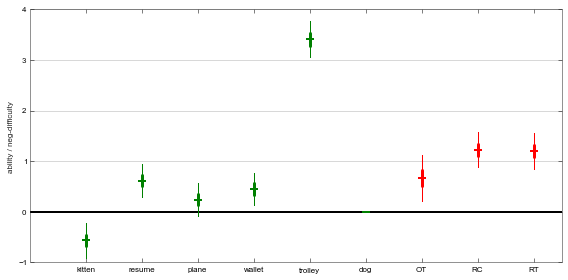These results confirm the original analysis. However, in this case, there can be no discussion of ceiling effects. Unlike with the raw score difference, the ceiling effect does not bias the the value towards zero but rather increase the uncertainty. We can demonstrate this by comparing the raw score difference with the logit score difference in a simulation.

Assume that the true values of $c$ are the ones we just estimated above. Assume that the true effect of the treatment is equal to the one measured in the original study $\delta_T-\delta_C = \delta_{OT}-\delta_{OC}=0.67$. We systematically vary the ability of the control group C. For each ability value, we simulate data from the model for single item and 200 subjects (100 in each group). We then estimate the $\delta_T-\delta_C$. We can then ask how does the magnitude of the base ability ($\delta_C$) influence the ability of our method to detect the effect size. In particular we are interested in cases where $\delta_C$ is very high (or very low).

First, generate data.

In :
def ordinalLogitERvs(beta, c,n,size=1):
''' generate data with expected response
frequency from n subjects
'''
# c must be strictly increasing
assert np.all(np.diff(c)>0)
def invLogit(x): return 1/(1+np.exp(-x))
p=+list(invLogit(beta-c))+
p=-np.diff(p)
#return np.random.multinomial(n,p,size)
return np.int32(np.round(p*n))
def reformatData(dat):
out=[]
for k in range(dat.size):
out.extend([k]*dat[k])
return np.array(out)
b=np.linspace(-10,7,51)
d=np.median(w['dd'][:,0])
c=np.median(w['c'],axis=0)
S=[];P=[]
for bb in b:
S.append([np.squeeze(ordinalLogitERvs(bb,c,100)),
np.squeeze(ordinalLogitERvs(bb+d,c,100))])
P.append([reformatData(S[-1]),
reformatData(S[-1])])
for k in range(b.size):
i1=np.nonzero(S[k]!=0)
i2=np.nonzero(S[k]!=0)
if max((S[k]!=0).sum(),(S[k]!=0).sum())<9:
s= max(min(i1,i2)-1,0)
e= min(max(i1[-1],i2[-1])+1,10)
S[k]=S[k][s:e+1]
S[k]=S[k][s:e+1]


Here we use a simplified model - one item, two groups.

In :
model='''
data {
int<lower=2> K;
int<lower=0> y1[K];// response control
int<lower=0> y2[K];// response treatment
}
parameters {
real<lower=-1000,upper=1000> d;
ordered[K-1] c;
}
model {
for (k in 1:(K-1)) c[k]~ uniform(-200,200);
for (k in 1:K){
for (n in 1:y1[k]) k~ ordered_logistic(0.0,c);
for (n in 1:y2[k]) k~ ordered_logistic(d  ,c);
}}
'''
sm9=pystan.StanModel(model_code=model)

INFO:pystan:COMPILING THE C++ CODE FOR MODEL anon_model_3863612723aa87f9526232c8bb8a40a0 NOW.


Run estimation. I will spare you the output here.

In [ ]:
ds=[];cs=[]
for k in range(len(S)):
if S[k].size==1: continue
dat = {'y1':S[k],'y2':S[k],'K':S[k].size}
fit = sm9.sampling(data=dat,iter=2000,
chains=4,thin=2,warmup=500,njobs=4,seed=4)
print fit
saveStanFit(fit,'dd%d'%k)

In :
ds=[];xs=[]
for k in range(b.size):
try:
xs.append(b[k])
ds.append(f)
except:pass
ds=np.array(ds);xs=np.array(xs)
res1=errorbar(ds.T,x=xs-0.1)
res2=np.zeros((b.size,5))
for k in range(b.size):
res2[k,:]=plotCIttest2(y1=P[k],
y2=P[k],x=b[k]+0.1)
plt.gca().set_visible(False)

<matplotlib.figure.Figure at 0x7f2a7ed5a350>
In :
d1=np.median(w['dd'][:,0])
d2=DS[DS[:,0]==1,-2].mean()-DS[DS[:,0]==0,-2].mean()
plt.figure(figsize=(8,4))
ax1=plt.gca()
plt.plot([-10,5],[d1,d1],'r')
plt.plot([-10,5],[0,0],'k')
temp=[list(xs)+list(xs)[::-1],
list(res1[:,1])+list(res1[:,2])[::-1]]
alpha=0.2,fc='k',ec='k'))
plt.plot(xs,res1[:,0],'k',alpha=0.4)
plt.ylim([-1.5,2])
plt.grid(b=False,axis='x')
plt.legend(['True ES','Estimate Ordinal Logit'],loc=8)
plt.ylabel('deltaT - deltaC')
plt.xlabel('deltaC')
plt.grid(b=False,axis='both')
ax2 = ax1.twinx()
temp=[list(b)+list(b)[::-1],
list(res2[:,1])+list(res2[:,2])[::-1]]
for t in range(len(temp))[::-1]:
if np.isnan(temp[t]):
temp.pop(t);temp.pop(t)
xy=np.array(temp).T,alpha=0.2,fc='m',ec='m'))
plt.plot(b,res2[:,0],'m')
plt.ylim([-1.5/d1*d2,2/d1*d2])
plt.xlim([-9,4.5])
plt.plot(-np.median(w['beta'],axis=0),[-0.3]*6,'+b',mew=2)
plt.plot(-np.median(w['beta']-np.atleast_2d(w['dd'][:,1]).T,
axis=0),[-0.5]*6,'+g',mew=2)
plt.legend(['Estimate T - C',
'Item Neg-Difficulty Original Study',
'Item Neg-Difficulty Replication'],loc=4)
plt.ylabel('T - C',color='m')
plt.grid(b=False,axis='both')
for tl in ax2.get_yticklabels():tl.set_color('m')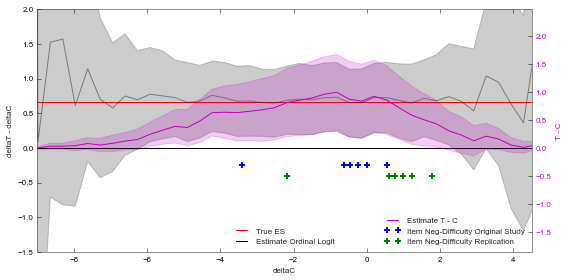The figure sums up this post. The estimates from the two methods (grey and magenta) share the y-axis, such that the vertical position of ground true treatment efect (red) and zero (black) is the same. The horizontal axis shows the ability of the control group ($\delta_C$). The ceiling effect is located on the right. Raw score difference (magenta) suffers from ceiling effect. To put the ceiling effects into context the blue and green circles show the neg-difficulty of each item in the original and replication study respectively. In this way we can say what kind of bias due to ceiling effect we would expect if we use items with the degree of difficulty observed in the original and replication study. The items in the original study (possibly with the exception of the kitten item) do not suffer from ceiling effects. However, several of the items in the replication study are located in the region with noticeable bias. At the same time it is clear that even if the replication study used the kitten item only, the ceiling effects would cut the effect size into half, but would not set it to zero. So ceiling effect yes, but not of a magnitude that could explain the zero raw score difference obtained in the replication.

However, this is not my main message. The figure shows that we should avoid the raw score difference in the first place. The magnitude of $T-C$ is biased towards zero. Even worse $T-C$ falsely claims high precision in the boundary regions - in the regions where the estimate is biased. In comparison, magnitude of $\delta_T-\delta_C$ is not biased by boundary effect. (The jumping around at the ends of the black line is due to discretization error introduced in the data generation proces.) Rather the boundary effect translates into worse precision of the estimate. Note also that the 95% interval of $\delta_T-\delta_C$ always includes the true effect size while $T-C$ incorrectly excludes it in the boundary regions.

What will you do next time when you analyse data where the values are constrained by boundaries? You can use some linear method such as the raw group difference or Anova and pray that your results are not be biased. This post showed you that you should fit a model from the logit family instead.